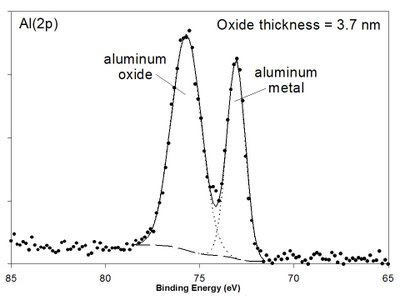### Aluminum Oxide Thickness Measurement

If the metal:oxide ratio can be determined for a thin film oxide sample (~0-9 nm) and if the inelastic mean free path (IMFP, λ) of the metal (λm) and oxide (λox) is known (or can be calculated), the oxide film thickness can be calculated using the calculations of the type developed by Strohmeier  and Carlson  defined as follows:

d=λoxsinθ ln(((NmλmIox)/(NoxλoxIm))+1) (Eq. 1)

where θ is the photoelectron take-off angle, Iox and Im are the area percentages of the oxide and metal peaks from the high-resolution spectrum, and Nm and Nox are the volume densities of the metal atoms in the metal and oxide, respectively.

For an aluminum oxide film on an aluminum substrate, the Al 2p spectrum has well separated oxide and metal peaks (example shown in Figure 1) and Io and Im values can be readily ascertained. Using the λ and N values proposed by Strohmeier , an Excel based aluminum oxide thickness calculator is presented. Just input the percentage of metal from the high-resolution spectrum to get a film thickness in Angstroms. (Note: you must download the file to Excel to use it - it is locked in Google Docs).Figure 1. Al 2p XPS spectrum of a thin film Al oxide on Al metal with a calculated oxide thickness of 3.7 nm.

References:
1. B.R. Strohmeier, Surf. Interface Anal. 15 (1990) 51.
2. T.A. Carlson, G.E. McGuire, J. Electron Spectrosc. Relat. Phenom, 1 (1972/73) 161.